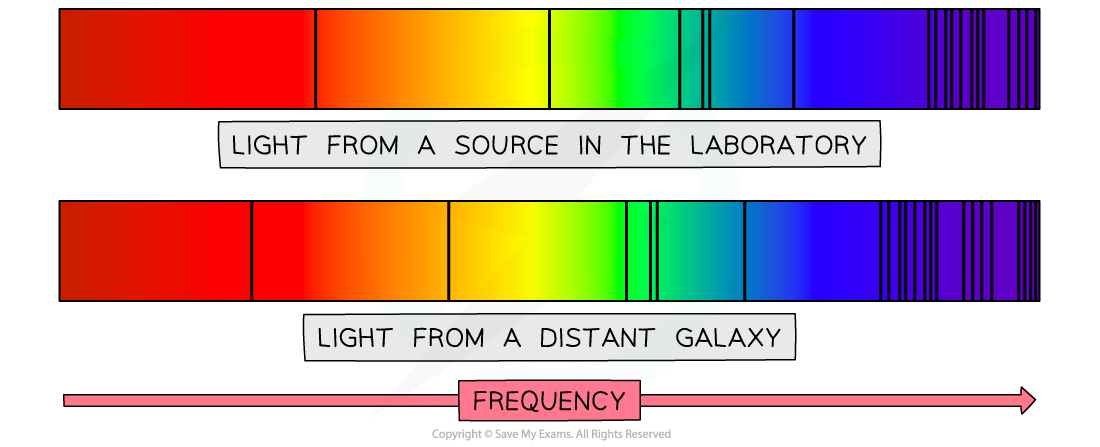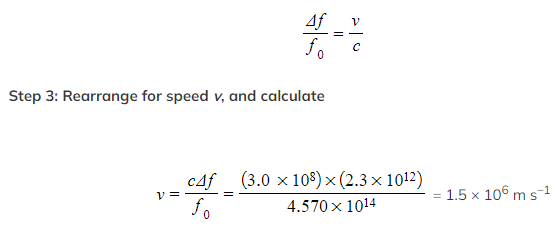# IB DP Physics: HL复习笔记9.5.2 Uses of The Doppler Effect

### Uses of The Doppler Effect

• The Doppler effect is important in many key areas of science including:
• Measuring the rate of blood flow within a patient
• Finding planet orbits around distant stars
• Mapping the expansion of the universe

• On Earth, the Doppler effect of sound can be easily observed when sound waves move past an observer at a notable speed
• In space, the Doppler effect of light can be observed when spectra of distant stars and galaxies are observed, this is known as:
• Redshift if the object is moving away from the Earth, or
• Blueshift if the object is moving towards the Earth
• Redshift is defined as:
The fractional increase in wavelength (or decrease in frequency) due to the source and observer receding from each other
• For non-relativistic galaxies, Doppler redshift can be calculated using:• Where:
• Δλ = shift in wavelength (m)
• λ = wavelength emitted from the source (m)
• Δf = shift in frequency (Hz)
• f = frequency emitted from the source (Hz)
• v = speed of recession (m s-1)
• c = speed of light in a vacuum (m s-1)

#### Worked Example

The spectra below show dark absorption lines against a continuous visible spectrum.A particle line in the spectrum of light from a source in the laboratory has a frequency of 4.570 × 1014 Hz. The same line in the spectrum of light from a distant galaxy has a frequency of 4.547 × 1014 Hz.

Calculate the speed of the distant galaxy in relation to the Earth. Determine whether it is moving towards or away from the Earth.

Step 1: Write down the known quantities
• Received frequency, fr = 4.547 × 1014 Hz
• Original frequency, f0 = 4.570 × 1014 Hz
• Shift in frequency, Δf = (4.547 – 4.570) × 1014 = –2.3 × 1012 Hz
• Speed of light, c = 3.0 × 108 m s–1
Step 2: Write down the Doppler redshift equationStep 4: Write a concluding sentence

The observed frequency is less than the emitted frequency (the light from a laboratory source), therefore, the source is receding, or moving away, from the Earth at 1.5 × 106 m s–1

#### An Expanding Universe

• After the discovery of Doppler redshift, astronomers began to realize that almost all the galaxies in the universe are receding
• This led to the idea that the space between the Earth and the galaxies must be expanding
• This expansion stretches out the light waves as they travel through space, shifting them towards the red end of the spectrum
• The more red-shifted the light from a galaxy is, the faster the galaxy is moving away from Earth• The expansion of the universe can be compared to dots on an inflating balloon
• As the balloon is inflated, the dots all move away from each other
• In the same way as the rubber stretches when the balloon is inflated, space itself is stretching out between galaxies
• Just like the dots, the galaxies move away from each other, however, they themselves do not move